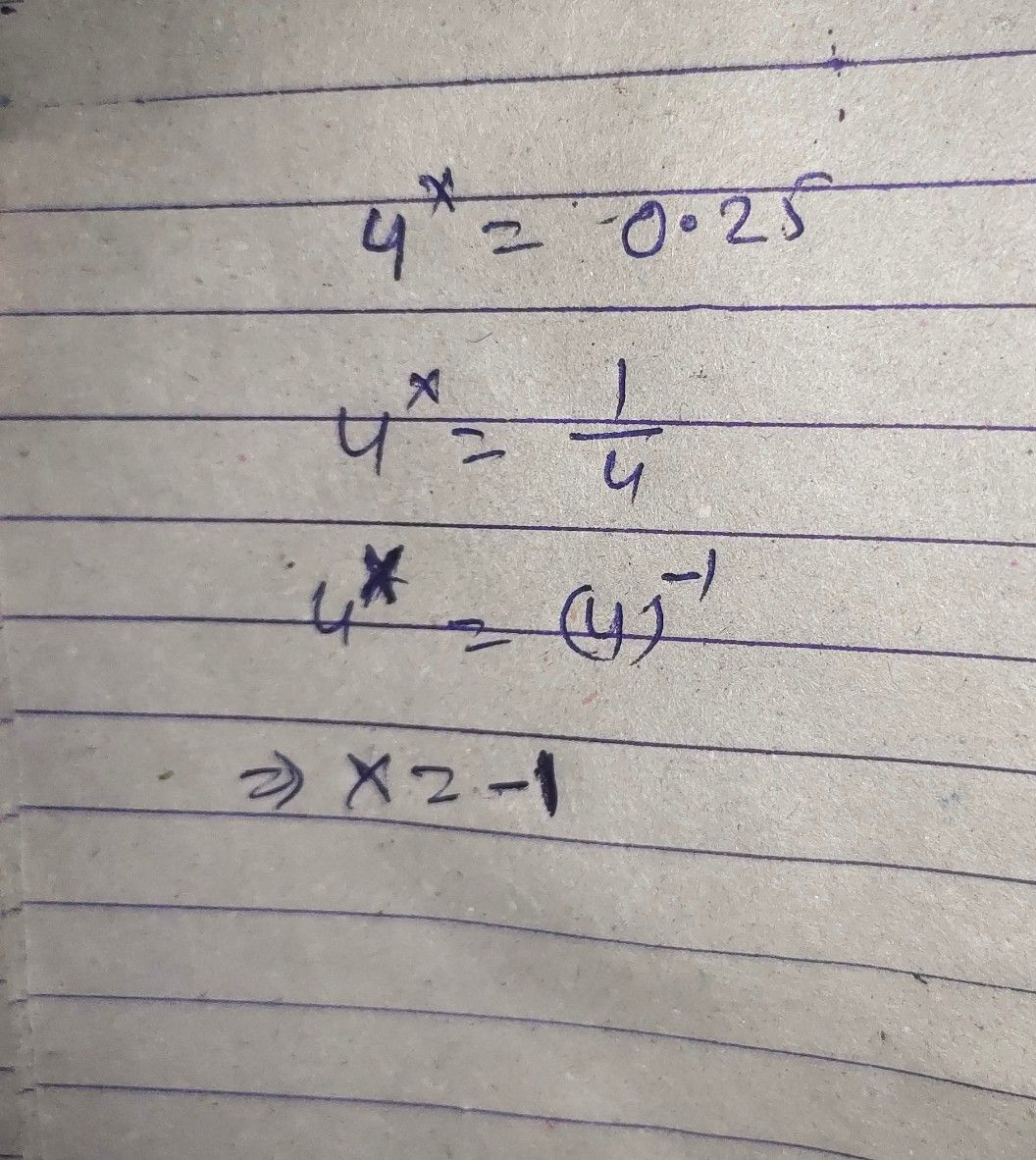Symbol
Problem$10y_{4}$ $0.2s=3$ $\leq 4$ $-$ $=$ $0.25$ find $w$
10th-13th grade
Algebra
SolutionQanda teacher - GodStudent
too late